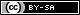# Dev Blog

 ./dev Original theme by orderedlist (CC-BY-SA) Where applicable, all content is licensed under a CC-BY-SA.# Misc. Math

## Landau Notation

$$f(x) = O(g(x)) \iff \limsup_{x \to \infty} \frac{f(x)}{g(x)} < \infty$$

$$f(x) = o(g(x)) \iff \lim_{x \to \infty} \frac{f(x)}{g(x)} = 0$$

$$f(x) = \Omega(g(x)) \iff \liminf_{x \to \infty} \frac{f(x)}{g(x)} > 0$$

$$f(x) = \omega(g(x)) \iff \liminf_{x \to \infty} \frac{f(x)}{g(x)} = \infty$$

$$f(x) = \Theta(g(x)) \iff f(x) = O(g(x)) \ \& \ f(x) = \Omega(g(x))$$

## $e$ is Irrational

Assume $e$ is rational. This means $e = \frac{p}{v}$.

We use the identity:

$$e = \sum_{k=0}^{\infty} \frac{1}{k!}$$

With the usual convention that $0!=1$.

$$\begin{array}{lcl} & e & = \frac{p}{v} \\ & & = \sum_{k=0}^{v} \frac{1}{k!} + \sum_{k=v+1}^{\infty} \frac{1}{k!} \\ \to & (v!) e & = (v-1)! p \\ & & = \sum_{k=0}^{v} \frac{v!}{k!} + ( \frac{1}{v+1} + \frac{1}{(v+1)(v+2)} + \cdots ) \end{array}$$

By assumption, $(v!) e = (v-1)! p \in \mathbb{Z}$. We also have the first sum $\sum_{k=0}^{v} \frac{v!}{k!} \in \mathbb{Z}$.

The second sum is greater than 0 and we can get bounds:

$$\begin{array}{lrcl} & 0 & < \frac{1}{v+1} + \frac{1}{(v+1)(v+2)} + \cdots & \le \frac{1}{v+1} \sum_{k=0}^{\infty} \frac{1}{(v+1)^k} \\ \to & 0 & < \frac{1}{v+1} + \frac{1}{(v+1)(v+2)} + \cdots & \le \frac{1}{v} \end{array}$$

Choose $v > 1$ and we have the second sum as non-integral, contradicting the assumption of rationality.